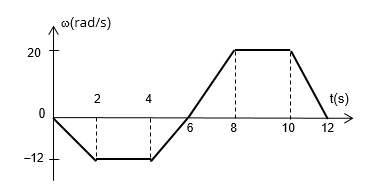# Consider the case of a rotating wheel at rest and starting asunshine022uv 2021-12-18 Answered

Consider the case of a rotating wheel at rest and starting a clockwise rotation, meaning the negative direction of the angular velocity, and increasing (negatively) its value up to $-12ra\frac{d}{\mathrm{sec}}$ for 2 seconds. It then maintains a constant velocity for 2 seconds, and then uniformly reduces the magnitude of the velocity for 2 seconds until the wheel is momentarily stopped and restarts its rotation counter clockwise with positive angular velocity, accelerating up to $20ra\frac{d}{\mathrm{sec}}$ in 2 seconds and remaining at a constant rotation for 2 more seconds. Finally, the wheel stops gradually in 2 seconds. Next you can see the graph of angular velocity versus time of this rotation:
$\overline{)\begin{array}{cc}\text{Slope:}& \\ \text{Origin intercept:}& \\ \text{Equation:}\omega =f\left(t\right)\end{array}}$You can still ask an expert for help

• Live experts 24/7
• Questions are typically answered in as fast as 30 minutes
• Personalized clear answers

Solve your problem for the price of one coffee

• Math expert for every subject
• Pay only if we can solve itambarakaq8

Step 1
The graph in the interval is a straight line
The standard equation of a line in slope intercept form is $y=mx+c$ where m is the slope of the line and c is the y-intercept.
The horizontal axis is x-axis and vertical axis is y-axis.
Step 2
The two points from which slope will be calculated are:
and
which are the respective endpoints of the line.
Calculate the slope.
$m=\frac{{y}_{2}-{y}_{1}}{{x}_{2}-{x}_{1}}$
$=\frac{-12-0}{2-0}$
$=-6$
Hence the slope is -6.
The y-intercept is the point where the line intersects the y-axis. This point is clearly the origin. Hence the origin y-intercept is 0 since the y-coordinate at this point is 0.
The y-axis is represented by $\omega$ and the x-axis is represented by
The equation of the line is thus framed.
$\omega =mt+c$
$\omega =-6t+0$
Hence the equation of the line is $\omega =-6t$

We have step-by-step solutions for your answer!usaho4w

Let us derive the formula to find the value of the slope if two points
and
on the straight line are known. Then we have
${y}_{1}=m{x}_{1}+b$
and ${y}_{2}=m{x}_{2}+b$
We know that, $\text{slope}=\frac{\text{change in y}}{\text{change in x}}$
Substituting the values of ${y}_{1}$ and ${a}_{2}$, we get
$\frac{{y}_{2}-{y}_{1}}{{x}_{2}-{x}_{1}}=\frac{\left(m{x}_{2}+b\right)-\left(m{x}_{1}+b\right)}{{x}_{2}-{x}_{1}}$
$=\frac{m{x}_{2}-m{x}_{1}}{{x}_{2}-{x}_{1}}$
$=\frac{m\left({x}_{2}-{x}_{1}\right)}{{x}_{2}-{x}_{1}}$
$=m$
Thus we find that the slope (m) is calculated as $\frac{\text{(change in y)}}{\text{(change in x)}}$
$m=\frac{\text{difference in y coordinates}}{\text{difference in x coordinates}}$

We have step-by-step solutions for your answer!